# Heat Capacity

The heat capacity measures the amount of heat necessary to raise the temperature of an object or system by one degree Celsius.

### Learning Objectives

Explain the enthalpy in a system with constant volume and pressure

### Key Takeaways

#### Key Points

• Heat capacity is the measurable physical quantity that characterizes the amount of heat required to change a substance’s temperature by a given amount. It is measured in joules per Kelvin and given by.
• The heat capacity is an extensive property, scaling with the size of the system.
• The heat capacity of most systems is not constant (though it can often be treated as such). It depends on the temperature, pressure, and volume of the system under consideration.

#### Key Terms

• heat capacity: The amount of heat energy needed to raise the temperature of an object or unit of matter by one degree Celsius; in units of joules per kelvin (J/K).
• enthalpy: the total amount of energy in a system, including both the internal energy and the energy needed to displace its environment

### Heat Capacity

Heat capacity (usually denoted by a capital C, often with subscripts), or thermal capacity, is the measurable physical quantity that characterizes the amount of heat required to change a substance’s temperature by a given amount. In SI units, heat capacity is expressed in units of joules per kelvin (J/K).

An object’s heat capacity (symbol C) is defined as the ratio of the amount of heat energy transferred to an object to the resulting increase in temperature of the object.

$\displaystyle{\text{C}=\frac{\text{Q}}{ \Delta \text{T}}.}$

Heat capacity is an extensive property, so it scales with the size of the system. A sample containing twice the amount of substance as another sample requires the transfer of twice as much heat (Q) to achieve the same change in temperature (ΔT). For example, if it takes 1,000 J to heat a block of iron, it would take 2,000 J to heat a second block of iron with twice the mass as the first.

### The Measurement of Heat Capacity

The heat capacity of most systems is not a constant. Rather, it depends on the state variables of the thermodynamic system under study. In particular, it is dependent on temperature itself, as well as on the pressure and the volume of the system, and the ways in which pressures and volumes have been allowed to change while the system has passed from one temperature to another. The reason for this is that pressure-volume work done to the system raises its temperature by a mechanism other than heating, while pressure-volume work done by the system absorbs heat without raising the system’s temperature. (The temperature dependence is why the definition a calorie is formally the energy needed to heat 1 g of water from 14.5 to 15.5 °C instead of generally by 1 °C. )

Different measurements of heat capacity can therefore be performed, most commonly at constant pressure and constant volume. The values thus measured are usually subscripted (by p and V, respectively) to indicate the definition. Gases and liquids are typically also measured at constant volume. Measurements under constant pressure produce larger values than those at constant volume because the constant pressure values also include heat energy that is used to do work to expand the substance against the constant pressure as its temperature increases. This difference is particularly notable in gases where values under constant pressure are typically 30% to 66.7% greater than those at constant volume.

### Thermodynamic Relations and Definition of Heat Capacity

The internal energy of a closed system changes either by adding heat to the system or by the system performing work. Recalling the first law of thermodynamics,

$\text{dU}=\delta \text{Q}-\delta \text{W}$.

For work as a result of an increase of the system volume we may write,

$\text{dU}=\delta \text{Q}-\text{PdV}$.

If the heat is added at constant volume, then the second term of this relation vanishes and one readily obtains

$\displaystyle{\left( \frac{\partial \text{U}}{\partial \text{T}}\right) _{\text{V}}=\left( \frac{\partial \text{Q}}{\partial \text{T}}\right) _{\text{V}}=\text{C}_{\text{V}}}$.

This defines the heat capacity at constant volume, CV. Another useful quantity is the heat capacity at constant pressure, CP. With the enthalpy of the system given by

$\text{H}=\text{U}+\text{PV}$,

our equation for dU changes to

$\text{dH}=\delta \text{Q}+\text{VdP}$,

and therefore, at constant pressure, we have

$(\frac{\partial \text{H}}{\partial \text{T}})_{\text{P}}=(\frac{\partial \text{Q}}{\partial \text{T}})_{\text{P}}=\text{C}_{\text{P}}$.

## Specific Heat

The specific heat is an intensive property that describes how much heat must be added to a particular substance to raise its temperature.

### Learning Objectives

Summarize the quantitative relationship between heat transfer and temperature change

### Key Takeaways

#### Key Points

• Unlike the total heat capacity, the specific heat capacity is independent of mass or volume. It describes how much heat must be added to a unit of mass of a given substance to raise its temperature by one degree Celsius. The units of specific heat capacity are J/(kg °C) or equivalently J/(kg K).
• The heat capacity and the specific heat are related by C=cm or c=C/m.
• The mass m, specific heat c, change in temperature ΔT, and heat added (or subtracted) Q are related by the equation: Q=mcΔT.
• Values of specific heat are dependent on the properties and phase of a given substance. Since they cannot be calculated easily, they are empirically measured and available for reference in tables.

#### Key Terms

• specific heat capacity: The amount of heat that must be added (or removed) from a unit mass of a substance to change its temperature by one degree Celsius. It is an intensive property.

### Specific Heat

The heat capacity is an extensive property that describes how much heat energy it takes to raise the temperature of a given system. However, it would be pretty inconvenient to measure the heat capacity of every unit of matter. What we want is an intensive property that depends only on the type and phase of a substance and can be applied to systems of arbitrary size. This quantity is known as the specific heat capacity (or simply, the specific heat), which is the heat capacity per unit mass of a material. Experiments show that the transferred heat depends on three factors: (1) The change in temperature, (2) the mass of the system, and (3) the substance and phase of the substance. The last two factors are encapsulated in the value of the specific heat.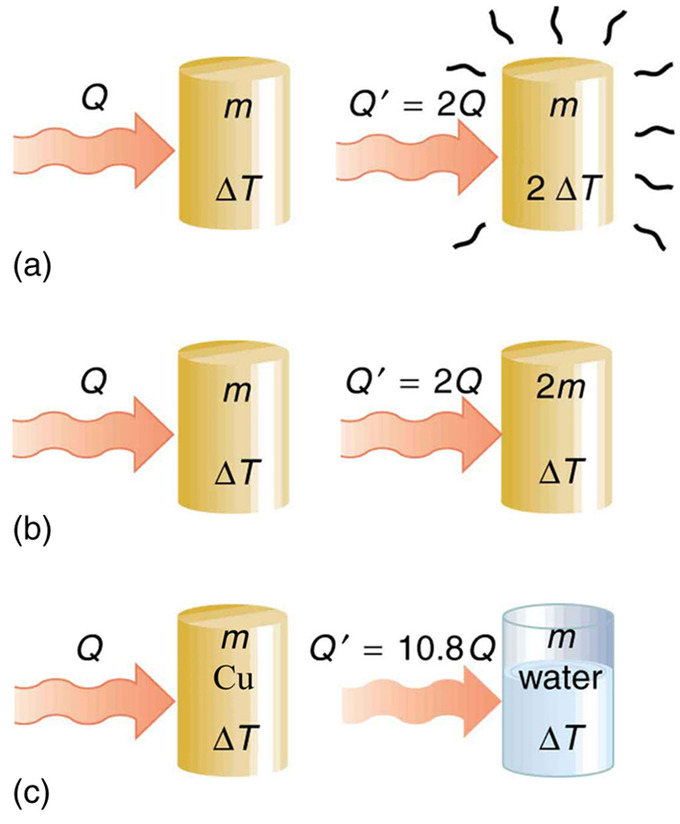Heat Transfer and Specific Heat Capacity: The heat Q transferred to cause a temperature change depends on the magnitude of the temperature change, the mass of the system, and the substance and phase involved. (a) The amount of heat transferred is directly proportional to the temperature change. To double the temperature change of a mass m, you need to add twice the heat. (b) The amount of heat transferred is also directly proportional to the mass. To cause an equivalent temperature change in a doubled mass, you need to add twice the heat. (c) The amount of heat transferred depends on the substance and its phase. If it takes an amount Q of heat to cause a temperature change ΔT in a given mass of copper, it will take 10.8 times that amount of heat to cause the equivalent temperature change in the same mass of water assuming no phase change in either substance.

Specific Heat Capacity: This lesson relates heat to a change in temperature. We discuss how the amount of heat needed for a temperature change is dependent on mass and the substance involved, and that relationship is represented by the specific heat capactiy of the substance, C.

The dependence on temperature change and mass are easily understood. Because the (average) kinetic energy of an atom or molecule is proportional to the absolute temperature, the internal energy of a system is proportional to the absolute temperature and the number of atoms or molecules. Since the transferred heat is equal to the change in the internal energy, the heat is proportional to the mass of the substance and the temperature change. The transferred heat also depends on the substance so that, for example, the heat necessary to raise the temperature is less for alcohol than for water. For the same substance, the transferred heat also depends on the phase (gas, liquid, or solid).

The quantitative relationship between heat transfer and temperature change contains all three factors:

$\text{Q}=\text{mc}\Delta \text{T}$,

where Q is the symbol for heat transfer, m is the mass of the substance, and ΔT is the change in temperature. The symbol c stands for specific heat and depends on the material and phase.

The specific heat is the amount of heat necessary to change the temperature of 1.00 kg of mass by 1.00ºC. The specific heat c is a property of the substance; its SI unit is J/(kg⋅K) or J/(kg⋅C). Recall that the temperature change (ΔT) is the same in units of kelvin and degrees Celsius. Note that the total heat capacity C is simply the product of the specific heat capacity c and the mass of the substance m, i.e.,

$\text{C}=\text{mc}$ or $\text{c}=\frac{\text{C}}{\text{m}}=\frac{\text{C}}{\rho \text{V}}$,

where ϱ is the density of the substance and V is its volume.

Values of specific heat must generally be looked up in tables, because there is no simple way to calculate them. Instead, they are measured empirically. In general, the specific heat also depends on the temperature. The table below lists representative values of specific heat for various substances. Except for gases, the temperature and volume dependence of the specific heat of most substances is weak. The specific heat of water is five times that of glass and ten times that of iron, which means that it takes five times as much heat to raise the temperature of water the same amount as for glass and ten times as much heat to raise the temperature of water as for iron. In fact, water has one of the largest specific heats of any material, which is important for sustaining life on Earth.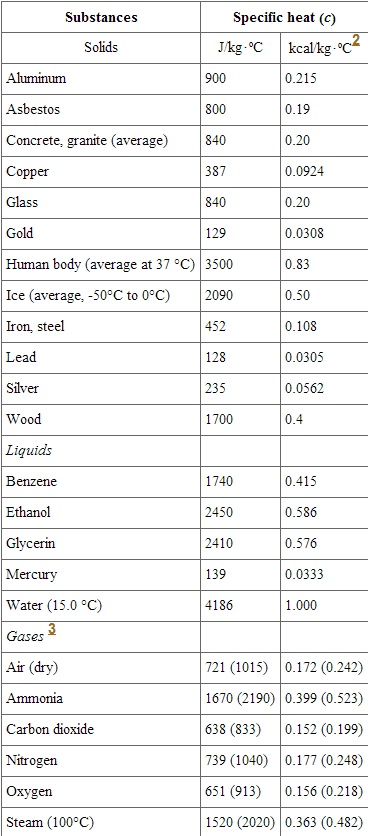Specific Heats: Listed are the specific heats of various substances. These values are identical in units of cal/(g⋅C).3. cv at constant volume and at 20.0ºC, except as noted, and at 1.00 atm average pressure. Values in parentheses are cp at a constant pressure of 1.00 atm.

## Calorimetry

Calorimetry is the measurement of the heat of chemical reactions or physical changes.

### Learning Objectives

Analyze the relationship between the gas constant for an ideal gas yield and volume

### Key Takeaways

#### Key Points

• A calorimeter is used to measure the heat generated (or absorbed) by a physical change or chemical reaction. The science of measuring these changes is known as calorimetry.
• In order to do calorimetry, it is crucial to know the specific heats of the substances being measured.
• Calorimetry can be performed under constant volume or constant pressure. The type of calculation done depends on the conditions of the experiment.

#### Key Terms

• constant-pressure calorimeter: An instrument used to measure the heat generated during changes that do not involve changes in pressure.
• calorimeter: An apparatus for measuring the heat generated or absorbed by either a chemical reaction, change of phase or some other physical change.
• constant-volume calorimeter: An instrument used to measure the heat generated during changes that do not involve changes in volume.

### Overview

Calorimetry is the science of measuring the heat of chemical reactions or physical changes. Calorimetry is performed with a calorimeter. A simple calorimeter just consists of a thermometer attached to a metal container full of water suspended above a combustion chamber. The word calorimetry is derived from the Latin word calor, meaning heat. Scottish physician and scientist Joseph Black, who was the first to recognize the distinction between heat and temperature, is said to be the founder of calorimetry.

Calorimetry requires that the material being heated have known thermal properties, i.e. specific heat capacities. The classical rule, recognized by Clausius and by Kelvin, is that the pressure exerted by the calorimetric material is fully and rapidly determined solely by its temperature and volume; this rule is for changes that do not involve phase change, such as melting of ice. There are many materials that do not comply with this rule, and for them, more complex equations are required than those below.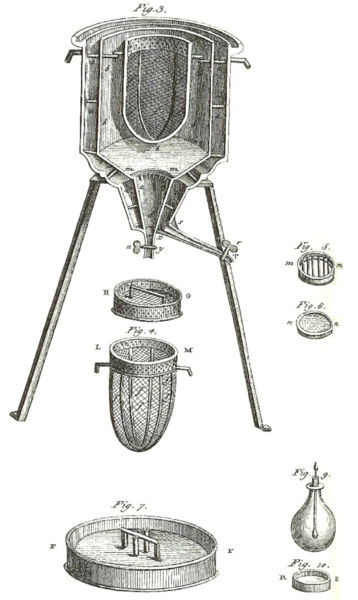Ice Calorimeter: The world’s first ice-calorimeter, used in the winter of 1782-83, by Antoine Lavoisier and Pierre-Simon Laplace, to determine the heat evolved in variouschemical changes; calculations which were based on Joseph Black’s prior discovery of latent heat. These experiments mark the foundation of thermochemistry.

### Basic Calorimetry at Constant Value

Constant-volume calorimetry is calorimetry performed at a constant volume. This involves the use of a constant-volume calorimeter (one type is called a Bomb calorimeter). For constant-volume calorimetry:

$\delta \text{Q}=\text{C}_{\text{V}}\Delta \text{T}=\text{mc}_{\text{V}}\Delta \text{T}$

where δQ is the increment of heat gained by the sample, CV is the heat capacity at constant volume, cv is the specific heat at constant volume, and ΔT is the change in temperature.

### Measuring Enthalpy Change

To find the enthalpy change per mass (or per mole) of a substance A in a reaction between two substances A and B, the substances are added to a calorimeter and the initial and final temperatures (before the reaction started and after it has finished) are noted. Multiplying the temperature change by the mass and specific heat capacities of the substances gives a value for the energy given off or absorbed during the reaction:

$\delta \text{Q}=\Delta \text{T}(\text{m}_{\text{A}}\text{c}_{\text{A}}+\text{m}_{\text{B}}\text{c}_{\text{B}})$

Dividing the energy change by how many grams (or moles) of A were present gives its enthalpy change of reaction. This method is used primarily in academic teaching as it describes the theory of calorimetry. It does not account for the heat loss through the container or the heat capacity of the thermometer and container itself. In addition, the object placed inside the calorimeter shows that the objects transferred their heat to the calorimeter and into the liquid, and the heat absorbed by the calorimeter and the liquid is equal to the heat given off by the metals.

### Constant-Pressure Calorimetry

A constant-pressure calorimeter measures the change in enthalpy of a reaction occurring in solution during which the atmospheric pressure remains constant. An example is a coffee-cup calorimeter, which is constructed from two nested Styrofoam cups and a lid with two holes, allowing insertion of a thermometer and a stirring rod. The inner cup holds a known amount of a solute, usually water, that absorbs the heat from the reaction. When the reaction occurs, the outer cup provides insulation. Then

$\text{C}_{\text{P}}=\frac{\text{W}\Delta \text{H}}{\text{M}\Delta \text{T}}$

where Cp is the specific heat at constant pressure, ΔH is the enthalpy of the solution, ΔT is the change in temperature, W is the mass of the solute, and M is the molecular mass of the solute. The measurement of heat using a simple calorimeter, like the coffee cup calorimeter, is an example of constant-pressure calorimetry, since the pressure (atmospheric pressure) remains constant during the process. Constant-pressure calorimetry is used in determining the changes in enthalpy occurring in solution. Under these conditions the change in enthalpy equals the heat (Q=ΔH).

## Specific Heat for an Ideal Gas at Constant Pressure and Volume

An ideal gas has different specific heat capacities under constant volume or constant pressure conditions.

### Learning Objectives

Explain how to derive the adiabatic index

### Key Takeaways

#### Key Points

• The specific heat at constant volume for a gas is given as $(\frac{\partial \text{U}}{\partial \text{T}})_{\text{V}}=\text{c}_{\text{v}}$.
• The specific heat at constant pressure for an ideal gas is given as $(\frac{\partial \text{H}}{\partial \text{T}})_{\text{V}}=\text{c}_{\text{p}}=\text{c}_{\text{v}}+\text{R}$.
• The heat capacity ratio (or adiabatic index ) is the ratio of the heat capacity at constant pressure to heat capacity at constant volume.

#### Key Terms

• Fundamental Thermodynamic Relation: In thermodynamics, the fundamental thermodynamic relation expresses an infinitesimal change in internal energy in terms of infinitesimal changes in entropy, and volume for a closed system in thermal equilibrium in the following way: dU=TdS-PdV. Here, U is internal energy, T is absolute temperature, S is entropy, P is pressure and V is volume.
• adiabatic index: The ratio of the heat capacity at constant pressure to heat capacity at constant volume.
• specific heat: The ratio of the amount of heat needed to raise the temperature of a unit mass of substance by a unit degree to the amount of heat needed to raise that of the same mass of water by the same amount.

### Specific Heat for an Ideal Gas at Constant Pressure and Volume

The heat capacity at constant volume of nR = 1 J·K−1 of any gas, including an ideal gas is:

$(\frac{\partial \text{U}}{\partial \text{T}})_{\text{V}}=\text{c}_{\text{v}}$

This represents the dimensionless heat capacity at constant volume; it is generally a function of temperature due to intermolecular forces. For moderate temperatures, the constant for a monoatomic gas is cv=3/2 while for a diatomic gas it is cv=5/2 (see ). Macroscopic measurements on heat capacity provide information on the microscopic structure of the molecules.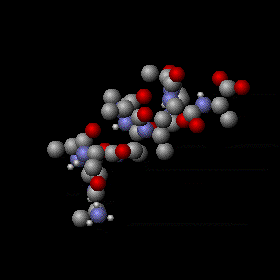Molecular internal vibrations: When a gas is heated, translational kientic energy of molecules in the gas will increase. In addition, molecules in the gas may pick up many characteristic internal vibrations. Potential energy stored in these internal degrees of freedom contributes to specific heat of the gas.

The heat capacity at constant pressure of 1 J·K−1 ideal gas is:

$(\frac{\partial \text{H}}{\partial \text{T}})_{\text{V}}=\text{c}_{\text{p}}=\text{c}_{\text{v}}+\text{R}$

where H=U+pV is the enthalpy of the gas.

Measuring the heat capacity at constant volume can be prohibitively difficult for liquids and solids. That is, small temperature changes typically require large pressures to maintain a liquid or solid at constant volume (this implies the containing vessel must be nearly rigid or at least very strong). It is easier to measure the heat capacity at constant pressure (allowing the material to expand or contract freely) and solve for the heat capacity at constant volume using mathematical relationships derived from the basic thermodynamic laws.

Utilizing the Fundamental Thermodynamic Relation we can show:

$\text{C}_{\text{p}}-\text{C}_{\text{V}}=\text{T}(\frac{\partial \text{P}}{\partial \text{T}})_{\text{V},\text{N}}(\frac{\partial \text{V}}{\partial \text{T}})_{\text{p},\text{N}}$

where the partial derivatives are taken at: constant volume and constant number of particles, and at constant pressure and constant number of particles, respectively.

The heat capacity ratio or adiabatic index is the ratio of the heat capacity at constant pressure to heat capacity at constant volume. It is sometimes also known as the isentropic expansion factor:

$\gamma =\frac{\text{C}_{\text{P}}}{\text{C}_{\text{V}}}=\frac{\text{c}_{\text{p}}}{\text{c}_{\text{v}}}$

For an ideal gas, evaluating the partial derivatives above according to the equation of state, where R is the gas constant for an ideal gas yields:

$\text{pV} = \text{RT}$

$\text{C}_{\text{p}}-\text{C}_{\text{V}}=\text{T}(\frac{\partial \text{P}}{\partial \text{T}})_{\text{V}}(\frac{\partial \text{V}}{\partial \text{T}})_{\text{p}}$

$\text{C}_{\text{p}}-\text{C}_{\text{V}}=-\text{T}(\frac{\partial \text{P}}{\partial \text{V}})_{\text{V}}(\frac{\partial \text{V}}{\partial \text{T}})_{\text{p}}^{2}$

$\text{P}=\frac{\text{RT}}{\text{V}}\text{n} \to (\frac{\partial \text{P}}{\partial \text{V}})_{\text{T}}=\frac{-\text{RT}}{\text{V}^{2}}=\frac{-\text{P}}{\text{V}}$

$\text{V}=\frac{\text{RT}}{\text{P}}\text{n} \to (\frac{\partial \text{V}}{\partial \text{T}})^{2}_{\text{p}}=\frac{\text{R}^{2}}{\text{P}^{2}}$

substituting:

$-\text{T}(\frac{\partial \text{P}}{\partial \text{V}})_{\text{V}}(\frac{\partial \text{V}}{\partial \text{T}})_{\text{p}}^{2}=-\text{T}\frac{-\text{P}}{\text{V}}\frac{\text{R}^{2}}{\text{P}^{2}}=\text{R}$

This equation reduces simply to what is known as Mayer’s relation: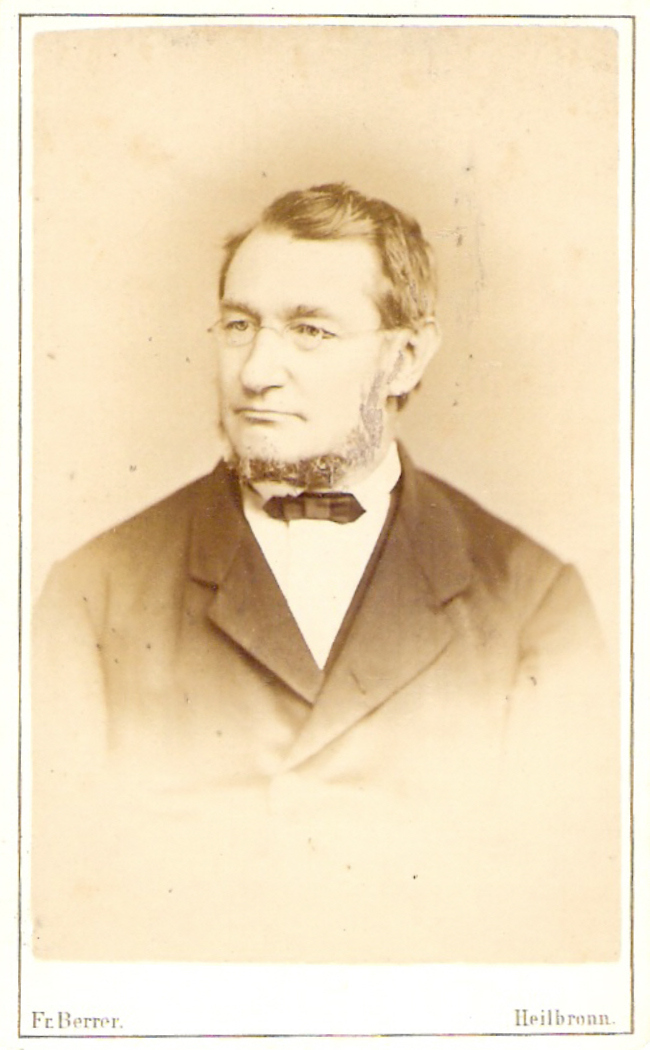Julius Robert Mayer: Julius Robert von Mayer (November 25, 1814 – March 20, 1878), a German physician and physicist, was one of the founders of thermodynamics. He is best known for his 1841 enunciation of one of the original statements of the conservation of energy (or what is now known as one of the first versions of the first law of thermodynamics): “Energy can be neither created nor destroyed. ” In 1842, Mayer described the vital chemical process now referred to as oxidation as the primary source of energy for any living creature. His achievements were overlooked and credit for the discovery of the mechanical equivalent of heat was attributed to James Joule in the following year. von Mayer also proposed that plants convert light into chemical energy.

$\text{C}_{\text{P}}-\text{C}_{\text{V}}=\text{R}$.

It is a simple equation relating the heat capacities under constant temperature and under constant pressure.

## Solving Problems with Calorimetry

Calorimetry is used to measure the amount of heat produced or consumed in a chemical reaction.

### Learning Objectives

Explain a bomb calorimeter is used to measure heat evolved in a combustion reaction

### Key Takeaways

#### Key Points

• Calorimetry is used to measure amounts of heat transferred to or from a substance.
• A calorimeter  is a device used to measure the amount of heat involved in a chemical or physical process.
• This means that the amount of heat produced or consumed in the reaction equals the amount of heat absorbed or lost by the solution.

#### Key Terms

• heat of reaction: The enthalpy change in a chemical reaction; the amount of heat that a systems gives up to its surroundings so it can return to its initial temperature.
• combustion: A process where two chemicals are combined to produce heat.

Calorimeters are designed to minimize energy exchange between the system being studied and its surroundings. They range from simple coffee cup calorimeters used by introductory chemistry students to sophisticated bomb calorimeters used to determine the energy content of food.

Calorimetry is used to measure amounts of heat transferred to or from a substance. To do so, the heat is exchanged with a calibrated object (calorimeter). The change in temperature of the measuring part of the calorimeter is converted into the amount of heat (since the previous calibration was used to establish its heat capacity ). The measurement of heat transfer using this approach requires the definition of a system (the substance or substances undergoing the chemical or physical change) and its surroundings (the other components of the measurement apparatus that serve to either provide heat to the system or absorb heat from the system). Knowledge of the heat capacity of the surroundings, and careful measurements of the masses of the system and surroundings and their temperatures before and after the process allows one to calculate the heat transferred as described in this section.

A calorimeter is a device used to measure the amount of heat involved in a chemical or physical process. For example, when an exothermic reaction occurs in solution in a calorimeter, the heat produced by the reaction is absorbed by the solution, which increases its temperature. When an endothermic reaction occurs, the heat required is absorbed from the thermal energy of the solution, which decreases its temperature. The temperature change, along with the specific heat and mass of the solution, can then be used to calculate the amount of heat involved in either case.

### Coffee-Cup Calorimeters

General chemistry students often use simple calorimeters constructed from polystyrene cups. These easy-to-use “coffee cup” calorimeters allow more heat exchange with their surroundings, and therefore produce less accurate energy values.

### Structure of the Constant Volume (or “Bomb”) Calorimeter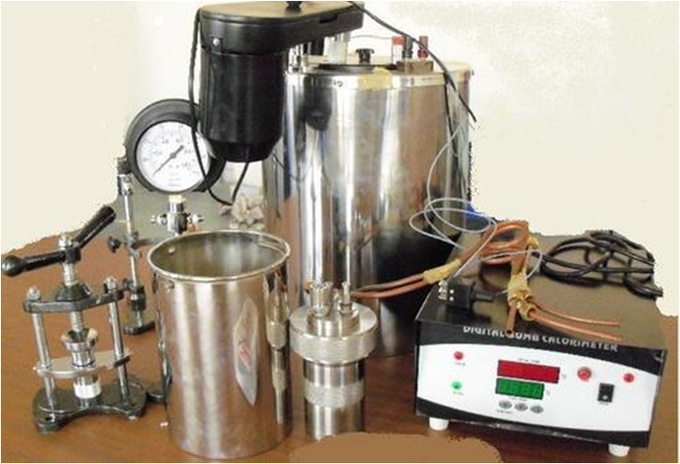Bomb Calorimeter: This is the picture of a typical setup of bomb calorimeter.

A different type of calorimeter that operates at constant volume, colloquially known as a bomb calorimeter, is used to measure the energy produced by reactions that yield large amounts of heat and gaseous products, such as combustion reactions. (The term “bomb” comes from the observation that these reactions can be vigorous enough to resemble explosions that would damage other calorimeters.) This type of calorimeter consists of a robust steel container (the “bomb”) that contains the reactants and is itself submerged in water. The sample is placed in the bomb, which is then filled with oxygen at high pressure. A small electrical spark is used to ignite the sample. The energy produced by the reaction is trapped in the steel bomb and the surrounding water. The temperature increase is measured and, along with the known heat capacity of the calorimeter, is used to calculate the energy produced by the reaction. Bomb calorimeters require calibration to determine the heat capacity of the calorimeter and ensure accurate results. The calibration is accomplished using a reaction with a known q, such as a measured quantity of benzoic acid ignited by a spark from a nickel fuse wire that is weighed before and after the reaction. The temperature change produced by the known reaction is used to determine the heat capacity of the calorimeter. The calibration is generally performed each time before the calorimeter is used to gather research data.

### Example: Identifying a Metal by Measuring Specific Heat

A 59.7 g piece of metal that had been submerged in boiling water was quickly transferred into 60.0 mL of water initially at 22.0 °C. The final temperature is 28.5 °C. Use these data to determine the specific heat of the metal. Use this result to identify the metal.

### Solution

Assuming perfect heat transfer, the heat given off by metal is the negative of the heat taken in by water, or:

$\text{q}_{\text{metal}}=-\text{q}_{\text{water}}$

In expanded form, this is:

$\text{c}_{\text{metal}} \times \text{m}_{\text{metal}} \times \left( \text{T}_{\text{f,metal}}-\text{T}_{\text{i,metal}} \right) = \text{c}_{\text{water}} \times \text{m}_{\text{water}} \times \left( \text{T}_{\text{f,water}}-\text{T}_{\text{i,water}} \right)$

Noting that since the metal was submerged in boiling water, its initial temperature was 100.0 °C; and that for water, 60.0 mL = 60.0 g; we have:

$\left( \text{c}_{\text{metal}} \right)\left( 59.7\text{ g} \right)\left( 28.5^{\text{o}} \text{C} - 100.0^{\text{o}} \text{C} \right) = \left( 4.18 \text{ J/g}^{\text{o}} \text{C} \right) \left( 60.0\text{ g} \right)\left( 28.5^{\text{o}} \text{C} - 22.0^{\text{o}} \text{C} \right)$

Solving this:

$\text{c}_{\text{metal}} = \dfrac{- \left( 4.184 \text{ J/g}^{\text{o}} \text{C} \right) \left( 60.0\text{ g} \right)\left( 6.5^{\text{o}} \text{C} \right)}{\left( 59.7\text{ g} \right)\left( -71.5^{\text{o}} \text{C} \right)} = 0.38 \text{ J/g}^{\text{o}} \text{C}$

Our experimental specific heat is closest to the value for copper (0.39 J/g °C), so we identify the metal as copper.